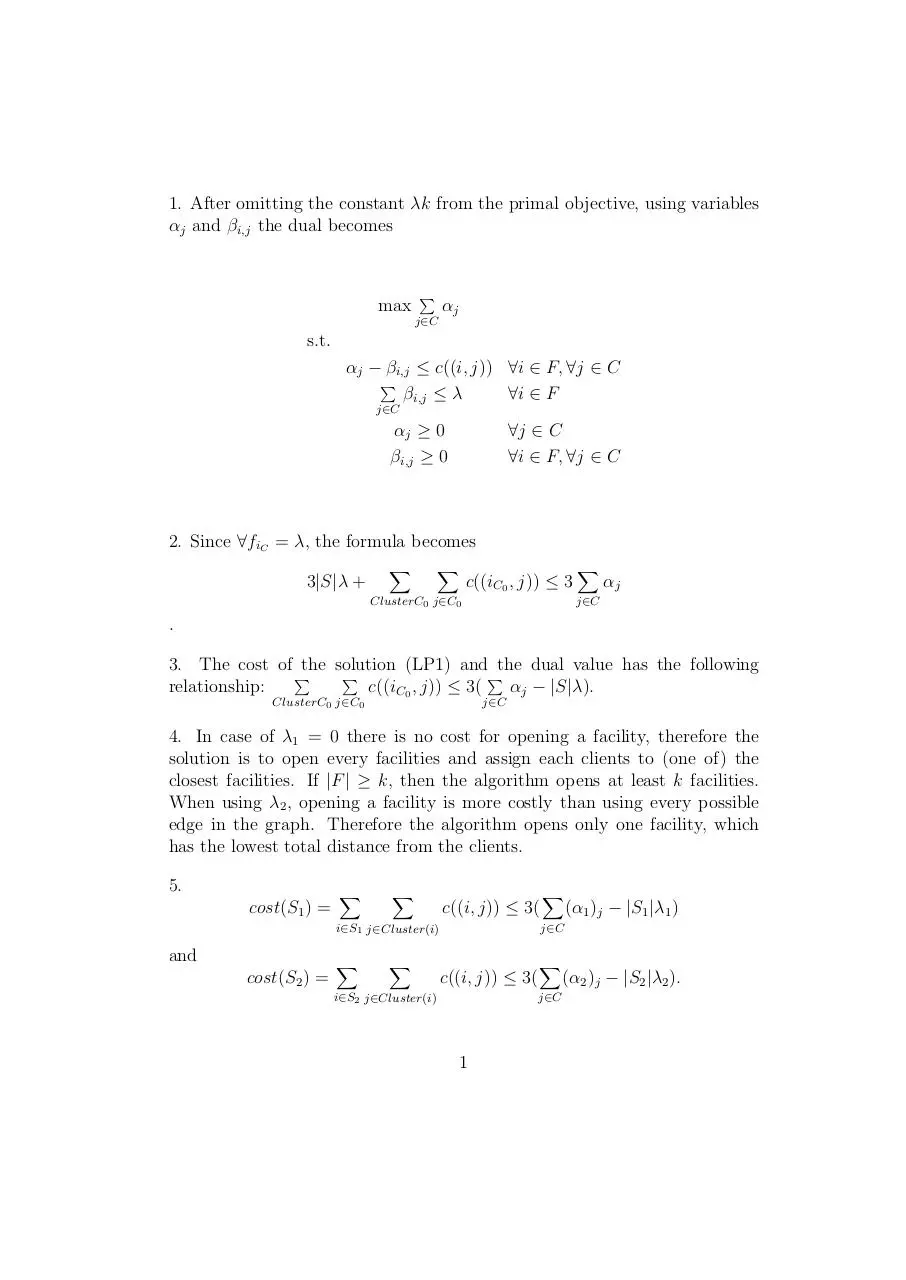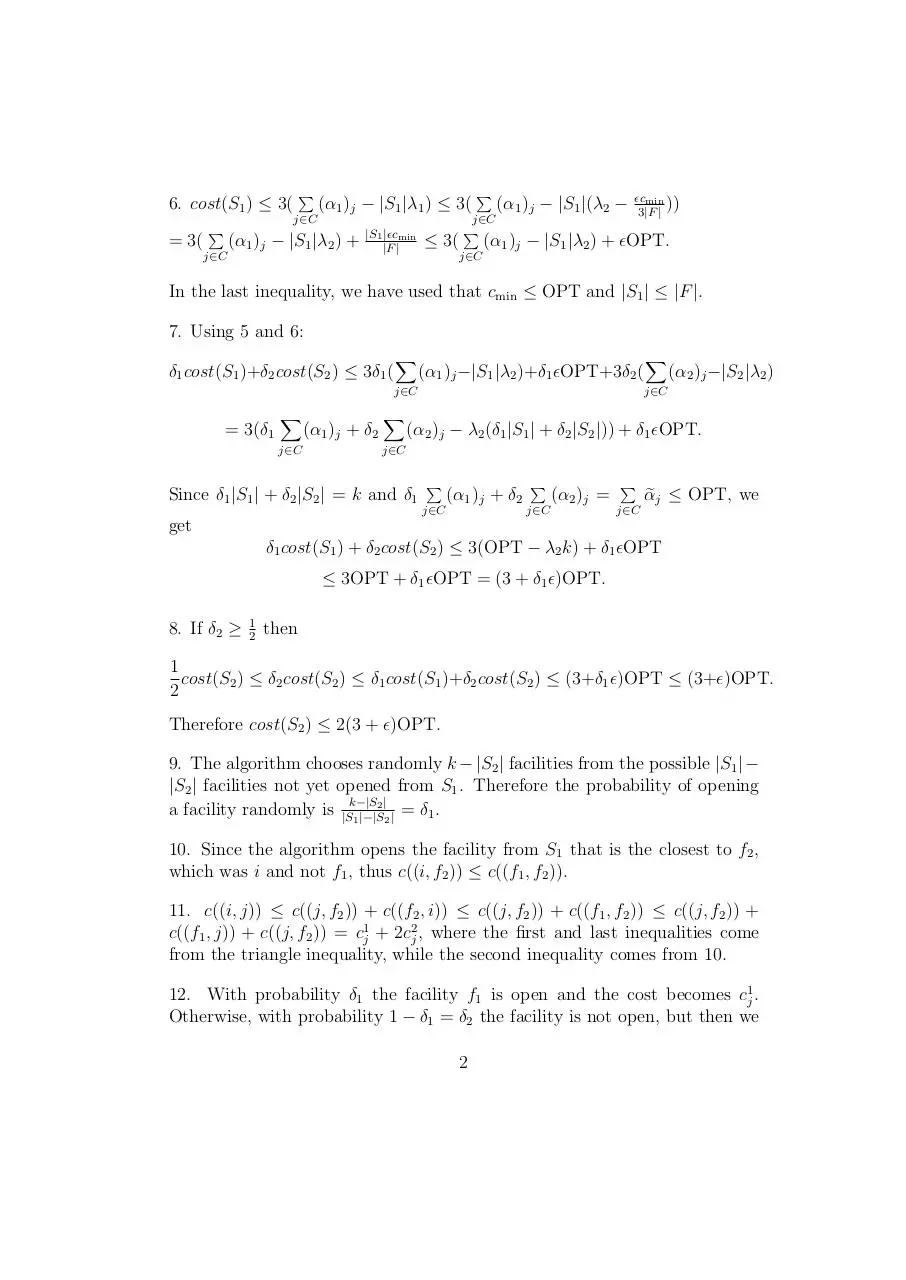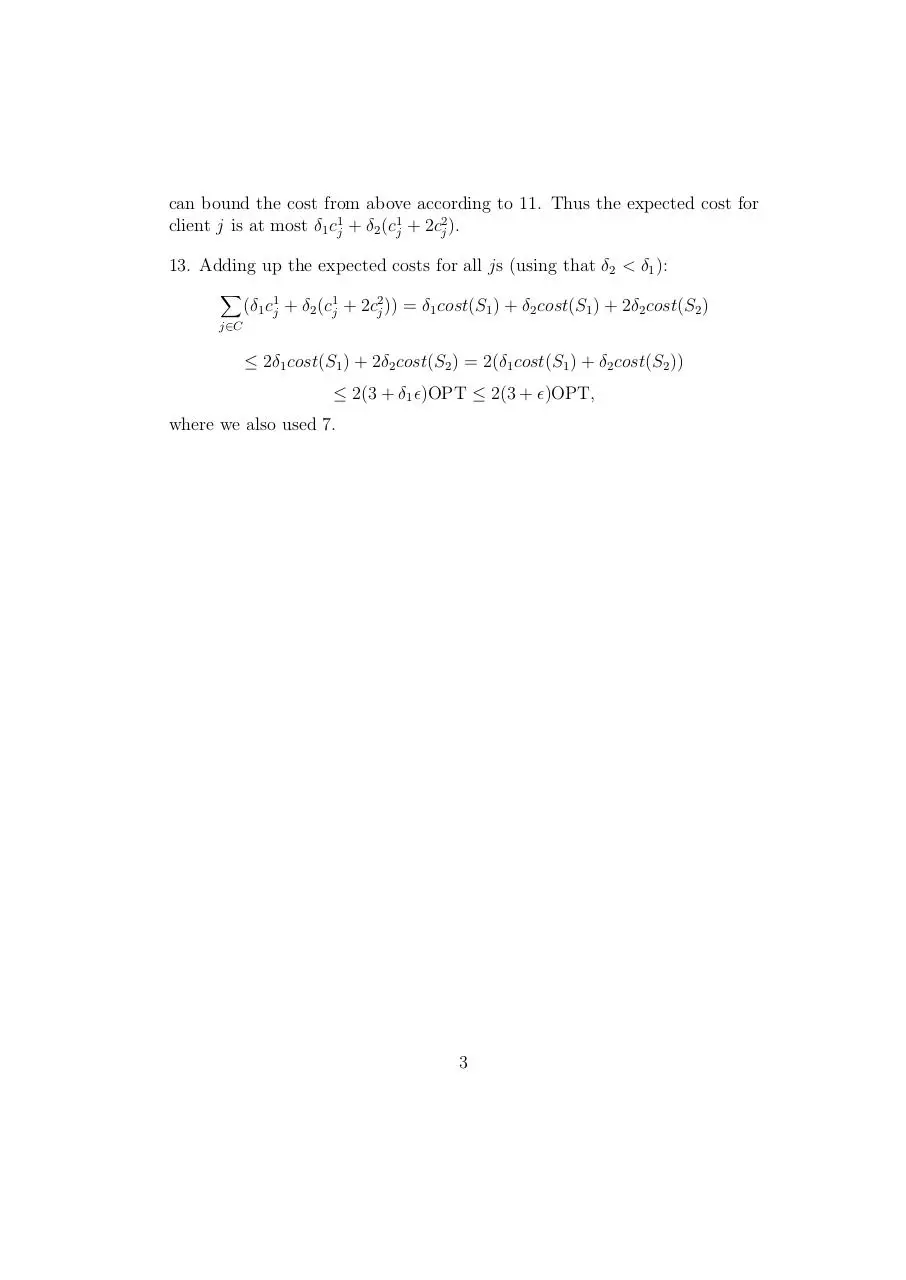# kmedian .pdf

### File information

This PDF 1.5 document has been generated by TeX / pdfTeX-1.40.14, and has been sent on pdf-archive.com on 19/02/2016 at 13:59, from IP address 195.111.x.x. The current document download page has been viewed 326 times.
File size: 92.26 KB (3 pages).
Privacy: public file

### Document preview

1. After omitting the constant λk from the primal objective, using variables
αj and βi,j the dual becomes

max

P

αj

j∈C

s.t.
αj − βi,j ≤ c((i, j)) ∀i ∈ F, ∀j ∈ C
P
βi,j ≤ λ
∀i ∈ F
j∈C

αj ≥ 0
βi,j ≥ 0

∀j ∈ C
∀i ∈ F, ∀j ∈ C

2. Since ∀fiC = λ, the formula becomes
3|S|λ +

X

X

c((iC0 , j)) ≤ 3

ClusterC0 j∈C0

X

αj

j∈C

.
3. The cost of the solution (LP1) and the dual value has the following
P
P
P
relationship:
αj − |S|λ).
c((iC0 , j)) ≤ 3(
ClusterC0 j∈C0

j∈C

4. In case of λ1 = 0 there is no cost for opening a facility, therefore the
solution is to open every facilities and assign each clients to (one of) the
closest facilities. If |F | ≥ k, then the algorithm opens at least k facilities.
When using λ2 , opening a facility is more costly than using every possible
edge in the graph. Therefore the algorithm opens only one facility, which
has the lowest total distance from the clients.
5.
cost(S1 ) =

X

X

c((i, j)) ≤ 3(

i∈S1 j∈Cluster(i)

X

(α1 )j − |S1 |λ1 )

j∈C

and
cost(S2 ) =

X

X

c((i, j)) ≤ 3(

i∈S2 j∈Cluster(i)

X

j∈C

1

(α2 )j − |S2 |λ2 ).

6. cost(S1 ) ≤ 3(

P

(α1 )j − |S1 |λ1 ) ≤ 3(

(α1 )j − |S1 |λ2 ) +

P

j∈C

(α1 )j − |S1 |(λ2 −

j∈C

j∈C

= 3(

P

|S1 |cmin
|F |

≤ 3(

P

cmin
))
3|F |

(α1 )j − |S1 |λ2 ) + OPT.

j∈C

In the last inequality, we have used that cmin ≤ OPT and |S1 | ≤ |F |.
7. Using 5 and 6:
δ1 cost(S1 )+δ2 cost(S2 ) ≤ 3δ1 (

X

(α1 )j −|S1 |λ2 )+δ1 OPT+3δ2 (

j∈C

= 3(δ1

X
j∈C

(α1 )j + δ2

X

X

(α2 )j −|S2 |λ2 )

j∈C

(α2 )j − λ2 (δ1 |S1 | + δ2 |S2 |)) + δ1 OPT.

j∈C

Since δ1 |S1 | + δ2 |S2 | = k and δ1

P

(α1 )j + δ2

j∈C

P

(α2 )j =

j∈C

P
j∈C

e j ≤ OPT, we
α

get
δ1 cost(S1 ) + δ2 cost(S2 ) ≤ 3(OPT − λ2 k) + δ1 OPT
≤ 3OPT + δ1 OPT = (3 + δ1 )OPT.
8. If δ2 ≥

1
2

then

1
cost(S2 ) ≤ δ2 cost(S2 ) ≤ δ1 cost(S1 )+δ2 cost(S2 ) ≤ (3+δ1 )OPT ≤ (3+)OPT.
2
Therefore cost(S2 ) ≤ 2(3 + )OPT.
9. The algorithm chooses randomly k − |S2 | facilities from the possible |S1 | −
|S2 | facilities not yet opened from S1 . Therefore the probability of opening
2|
a facility randomly is |Sk−|S
= δ1 .
1 |−|S2 |
10. Since the algorithm opens the facility from S1 that is the closest to f2 ,
which was i and not f1 , thus c((i, f2 )) ≤ c((f1 , f2 )).
11. c((i, j)) ≤ c((j, f2 )) + c((f2 , i)) ≤ c((j, f2 )) + c((f1 , f2 )) ≤ c((j, f2 )) +
c((f1 , j)) + c((j, f2 )) = c1j + 2c2j , where the first and last inequalities come
from the triangle inequality, while the second inequality comes from 10.
12. With probability δ1 the facility f1 is open and the cost becomes c1j .
Otherwise, with probability 1 − δ1 = δ2 the facility is not open, but then we
2

can bound the cost from above according to 11. Thus the expected cost for
client j is at most δ1 c1j + δ2 (c1j + 2c2j ).
13. Adding up the expected costs for all js (using that δ2 &lt; δ1 ):
X

(δ1 c1j + δ2 (c1j + 2c2j )) = δ1 cost(S1 ) + δ2 cost(S1 ) + 2δ2 cost(S2 )

j∈C

≤ 2δ1 cost(S1 ) + 2δ2 cost(S2 ) = 2(δ1 cost(S1 ) + δ2 cost(S2 ))
≤ 2(3 + δ1 )OPT ≤ 2(3 + )OPT,
where we also used 7.

3kmedian.pdf (PDF, 92.26 KB)

### Share on social networks

#### HTML Code

Copy the following HTML code to share your document on a Website or Blog